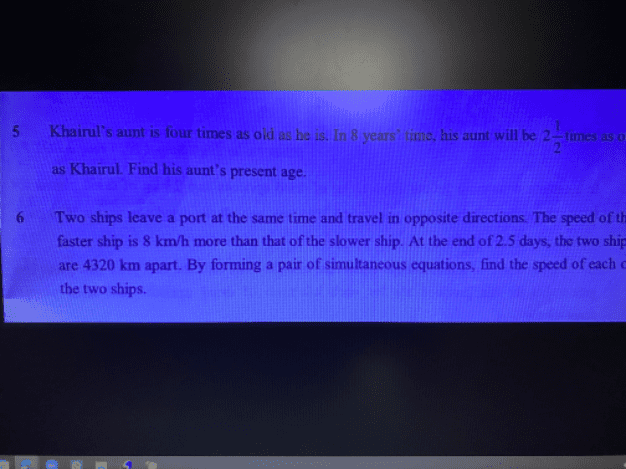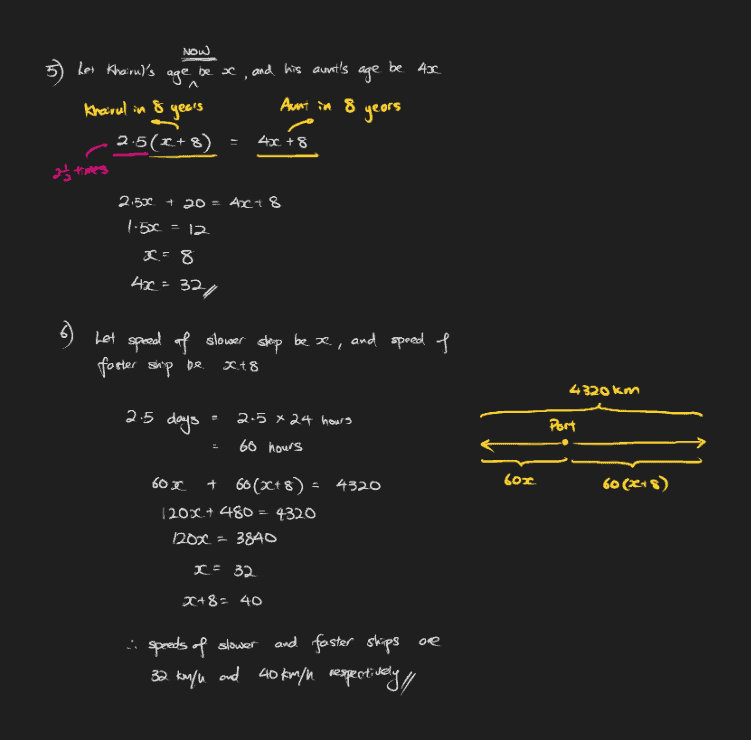# QuestionLet A be the aunt’s current age.
Let K be Khairul’s current age.

A = 4K … (1)

A + 8 = 2.5(K + 8)
A + 8 = 2.5K + 20
A = 2.5K + 12 … (2)

Substituting (1) into (2):
4K = 2.5K + 12
1.5K = 12
K = 8
So A = 4K =4 * 8 = 32 #

0 Replies 1 Like0 Replies 1 Like